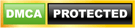# Case Statement/ Expression in SQL server

Sometimes we need to get one result output from multiple possible outcomes based on the evaluation of some expression. This expression can be a simple case expression which compares an expression with a set of Expression/Values to determine the result or it can be a search case expression where it evaluates a list of Boolean expressions to determine the result.

We can use the case statement in Select statement, Update statement, in Where clause, In clause, Having Clause, in  procedures and in User Defined Functions

Simple Case expression:-It compares an expression with a set of Expression/Values to determine the result.

Syntax:-

CASE input_expression
WHEN when input_expression 1 THEN result_expression1
WHEN when input_expression 2 THEN result_expression2 [ …n ]
ELSE else_result_expression
END

Example :-Suppose we have a stored procedure ‘usp_getcountryname’ which takes country code as input and returns the country name as output.

Create Procedure usp_getcountryname
(@I_countrycode nvarchar(10))

As
Begin

select case (@I_countrycode)
when ‘IN ‘ then ‘INDIA’
when ‘HK ‘ then ‘HONG KONG’
when ‘CN ‘ then ‘CHINA’
when ‘BR ‘ then ‘BRAZIL’
when ‘AU ‘ then ‘AUSTRALIA’
when ‘AF ‘ then ‘AFGHANISTAN’
else ‘No country found’   end as Countryname

End

when we execute this stored procedure using the command given below, it gives the result  ‘INDIA’ . In the above example, we compare the input value with multiple values and based upon the comparison , it gives the result.

exec usp_getcountryname ‘IN’

Search case Expression:- In this case, it evaluates a list of Boolean expressions to determine the result.

Syntax:-
CASE
WHEN Boolean_expression1 THEN expression1
WHEN Boolean_expression2 THEN expression2
WHEN Boolean_expression2 THEN expression2 […n]
ELSE expression
END

Example:- Suppose we have a table called tbl_Employee which contains the employee information regarding  their name, gender, age and salary.

Table creation script is given below:-

create table tbl_Employee(id int identity(1,1),Empname nvarchar(100),Gender nvarchar(5),Age int,Salary int)

Use the following Insert script to insert data in the table mentioned above:-

insert into tbl_Employee(Empname,Gender,Age,Salary)

select ‘Ram’,’M’,20,100000

union all

select ‘Neha’,’F’, 25,200000

union all

select ‘Praveen’,’M’,25,200000

union all

select ‘Garima’,’F’, 20, 100000

So, the initial data is given below:-

Select * from tbl_Employee

Suppose we have a requirement to update the salary of employees based on the following given conditions :-

1) If female and age less than 25 increase salary by 4 time
2) If female and age is greater or equal to 25 then increase salary by 3 times
3) If male and age is less than 25 then increase salary by 4.5 times
4) If male and age is greater or equal to 25 then increase salary by 2.5

In that case we can use the Search case expression to update employee salary

After the execution of the above update command, the data in the table becomes

Summary:- In this article , I tried to explain the Case expresion concept in SQL server with the help of examples.1.MY MONEYWORLD says: